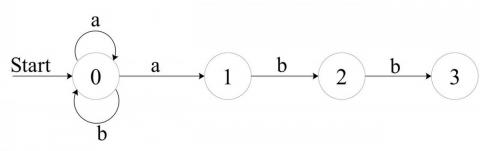## 正则表达式匹配和 NFA/DFA

2014-01-04

``````isMatch("aa","a")       → false
isMatch("aa","aa")      → true
isMatch("aaa","aa")     → false
isMatch("aa", "a*")     → true
isMatch("aa", ".*")     → true
isMatch("ab", ".*")     → true
isMatch("aab", "c*a*b") → true``````

## DFA 和 NFA 的概念nfadfa

DFA 的状态数目和 NFA 一样，但是一般实践过程中 DFA 的状态转移要多，所以 DFA 相对来说要难构造一些，同时 DFA 比 NFA 需要的内存空间更大。正因为在 NFA 中一个状态可能向多个状态转移，在极端的情况下其效率比不过 DFA。更多关于正则分类可以参考正则表达式引擎及其分类

## 一个尝试实现

``````enum OpType {
ZERO_PLUS_ONE,
ANY_ONE,
MUST_ONE
};
struct State {
OpType         type;
int            id;
char           value;
bool           end;
State*         prev;
vector<State*> next;

State(OpType t, int i, char v, State *p) :
type(t), id(i), value(v), end(false), prev(p) {
if(type == ZERO_PLUS_ONE)
next.push_back(this);
if(p == NULL)
prev = this;
}

next.push_back(n);
// 匹配任意，前驱加上当前需要添加的状态
if(type == ZERO_PLUS_ONE && prev != NULL)
}
};``````

``````State* construct_dfa(const char* pattern) {
if(pattern == NULL) return NULL;
const char* p = pattern;
State* start = new State(ANY_ONE, Num, '.', NULL);
State* cur   = start;
State* next  = NULL;
char   prev  = '.';
Num = 1;
while(*p && *p != '\0') {
if(*(p+1) != '*') {
OpType type;
char value;
if(*p == '*') {
type = ZERO_PLUS_ONE; //匹配 0 个或者多个
value = prev;
} else {
value = *p;
type = *p == '.'? ANY_ONE : MUST_ONE; //匹配任意一个。或者指定的字符
}
next = new State(type, Num, value, cur);
prev = *p, p++;
} else {
next = new State(ZERO_PLUS_ONE, Num, *p, cur);
prev = '*', p+=2;
}
cur = next;
Num++;
}
cur->end = true;
// 例如  ab*a*c*  对于 "a"，即使后面几个*, "a"也算是一个 end，
while(cur->type == ZERO_PLUS_ONE) {
cur = cur->prev;
cur->end = true;
}
return start;
}``````